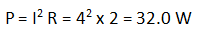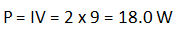# Question Guide – DC Circuit

This question is related to the topic “DC Circuit” in O Level Physics tuition.

Question: A resistor of resistance 2.0 Ω is connected across a series of cells of e.m.f. 9.0 V by wires of negligible resistance. A current 4.0 A passes through the resistor. Calculate (a) The power dissipated in the resistor, (b) The total power produced by the cells, (c) State a reason for the difference in values in (a) and (b).
(a) The power dissipated in the resistor, Solution:(b) The total power produced by the cells, Solution:(c) State a reason for the difference in values in (a) and (b). Observation: If total resistance is just 2 Ω, circuit currentBut the actual current is lower i.e. 4.0 A. This shows that the
total circuit resistance is actually higher than 2 Ω.
Typically, the cells have significant internal resistance. Thus, the higher circuit resistance. Solution: Some power is dissipated in the internal resistance of the cells.

If you need help in pure physics and you are taking the O level examination for this subject, why not join our physics tuition classes.

WhatsApp 8201 5955 to register now.

Sample Question and Solution on DC Circuit ultima modifica: 2017-10-18T07:10:35+00:00 da